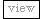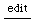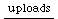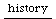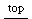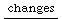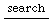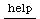## Computing exponential curve factor given midpoint y value

The question was raised on the sc-users list how to calculate the curve factor for a ControlSpec or envelope segment, given 3 values:

(Here, considering mapping a [0..1] value by a ControlSpec, c)

y1 = c.map(0) – i.e., minval in the ControlSpec
y = c.map(0.5)
y2 = c.map(1) – i.e., maxval in the ControlSpec

If c = ControlSpec(0, 1, -2) (curve factor = -2), c.map(0.5) = 0.73105857863. What was desired is a way to calculate the curve factor given y1 = 0, y2 = 1, and y = 0.73105857863.

The way the curve factor is incorporated into the CurveWarp formula is not very obvious, but eventually it can be reduced to a quadratic equation. This function uses the quadratic formula to solve that equation for any y1, y and y2 where y1 < y < y2.

Some additional code had to be added because of numeric instability just above the midpoint between y1 and y2. If y < ((y1 + y2) * 0.5), everything works. Just slightly above, and the result can't be trusted. So the function compensates by mirroring values above the 2-point mean to the respective position below.

f = { |minval, midval, maxval|
var a, b, c, sqrterm, qresult, sgn = sign(maxval - minval);
// the formula is unstable just above the average of minval and maxval
// so mirror the midval around the average
(midval > ((maxval + minval) * 0.5)).if({
midval = minval + maxval - midval;
sgn = sgn.neg;
});
a = midval - minval;
b = minval - maxval;
c = maxval - midval;
sqrterm = sqrt(b.squared - (4 * a * c));
(((qresult = (sqrterm - b) / (2 * a))).abs != 1).if({
log(qresult.squared).abs * sgn
}, {
log(((b.neg - sqrterm) / (2 * a)).squared).abs * sgn
});
};

f.(0, 0.73105857863, 1) == -2

hjh